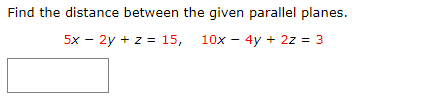Home / Answered Questions / Other / find-the-distance-between-the-given-parallel-planes-5x-2y-z-15-10x-4y-2z-3-aw994

# (Solved): Find The Distance Between The Given Parallel Planes. 5x â€“ 2y + Z = 15, 10x â€“ 4y + 2z = 3...Find the distance between the given parallel planes. 5x â€“ 2y + z = 15, 10x â€“ 4y + 2z = 3

We have an Answer from Expert